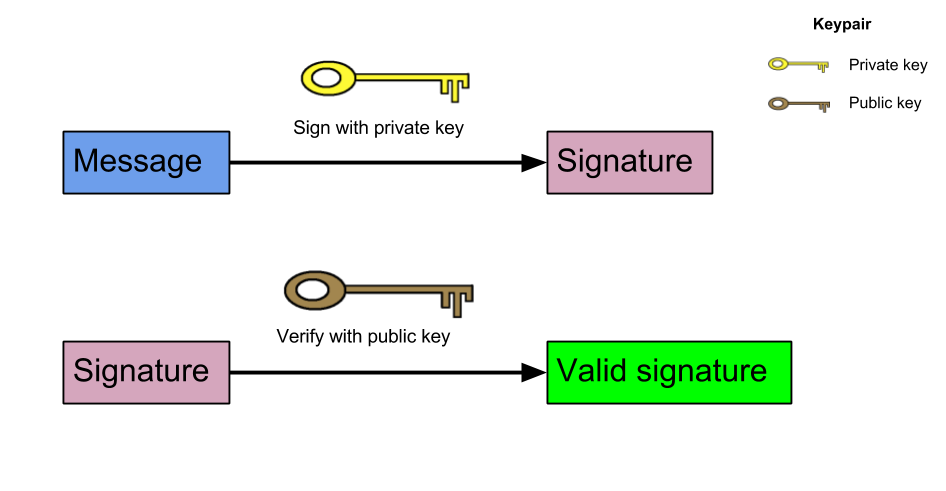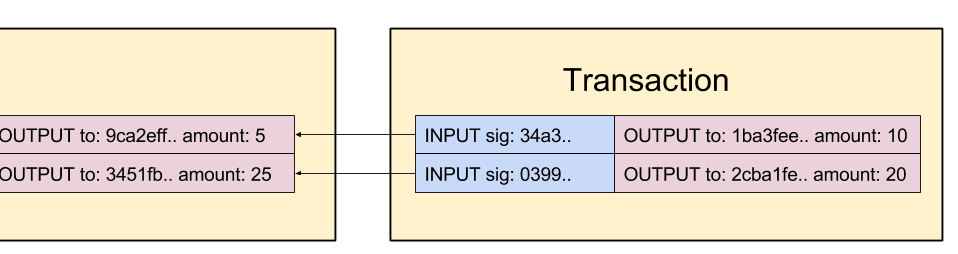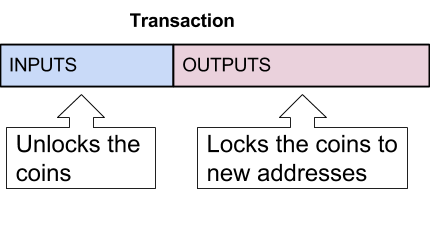# 03. Transactions

## Overview

In this chapter we will introduce the concept of transactions. With this modification, we actually shift from our project from a “general purpose” blockchain to a cryptocurrency. As a result, we can send coins to addresses if we can show a proof that we own them in the first place.

To enable all this, a lot of new concepts must presented. This includes public-key cryptography, signatures and transactions inputs and outputs.

This chapter has been copied from the original Naivecoin tutorial made by Lauri Hartikka and adapted for the Proof of Stake consensus. See the original page here: https://lhartikk.github.io/jekyll/update/2017/07/12/chapter3.html

## Public-key cryptography and signatures

In Public-key cryptography you have a keypair: a secret key and a public key. The public key can be derived from the secret key, but the secret key cannot be derived from the public key. The public key (as the name implies) can be shared safely to anyone.

Any messages can be signed using the private key to create a signature. With this signature and the corresponding public key, anyone can verify that the signature is produced by the private key the that matches the public key.We will use a library called elliptic for the public-key cryptography, which uses elliptic curves. (= ECDSA)

Conclusively, two different cryptographic functions are used for different purposes in the cryptocurrency:

• Hash function (SHA256) for the Proof-of-work mining (The hash is also used to preserve block integrity)
• Public-key cryptography (ECDSA) for transactions (we’ll be implementing in this chapter )

## Private-keys and public keys (in ECDSA)

A valid private key is any random 32 byte string, eg. `19f128debc1b9122da0635954488b208b829879cf13b3d6cac5d1260c0fd967c`

A valid public key is ‘04’ concatenated with a 64 byte string, e.g `04bfcab8722991ae774db48f934ca79cfb7dd991229153b9f732ba5334aafcd8e7266e47076996b55a14bf9913ee3145ce0cfc1372ada8ada74bd287450313534a`

The public key can be derived from the private key. The public-key will be used as the ‘receiver’ (= address) of the coins in a transaction.

## Transactions overview

Before writing any code, let’s get an overview about the structure of transactions. Transactions consists of two components: inputs and outputs. Outputs specify where the coins are sent and inputs give a proof that the coins that are actually sent exists in the first place and are owned by the “sender”. Inputs always refer to an existing (unspent) output.## Transaction outputs

Transaction outputs (txOut) consists of an address and an amount of coins. The address is an ECDSA public-key. This means that the user having the private-key of the referenced public-key (=address) will be able to access the coins.

``````class TxOut {
public amount: number;

constructor(address: string, amount: number) {
this.amount = amount;
}
}
``````

## Transaction inputs

Transaction inputs (txIn) provide the information “where” the coins are coming from. Each txIn refer to an earlier output, from which the coins are ‘unlocked’, with the signature. These unlocked coins are now ‘available’ for the txOuts. The signature gives proof that only the user, that has the private-key of the referred public-key ( =address) could have created the transaction.

``````class TxIn {
public txOutId: string;
public txOutIndex: number;
public signature: string;
}
``````

It should be noted that the txIn contains only the signature (created by the private-key), never the private-key itself. The blockchain contains public-keys and signatures, never private-keys.

As a conclusion, it can also be thought that the txIns unlock the coins and the txOuts ‘relock’ the coins:## Transaction structure

The transactions structure itself is quite simple as we have now defined txIns and txOuts.

``````class Transaction {
public id: string;
public txIns: TxIn[];
public txOuts: TxOut[];
}
``````

## Transaction id

The transaction id is calculated by taking a hash from the contents of the transaction. However, the signatures of the txIds are not included in the transaction hash as the will be added later on to the transaction.

``````const getTransactionId = (transaction: Transaction): string => {
const txInContent: string = transaction.txIns
.map((txIn: TxIn) => txIn.txOutId + txIn.txOutIndex)
.reduce((a, b) => a + b, '');

const txOutContent: string = transaction.txOuts
.map((txOut: TxOut) => txOut.address + txOut.amount)
.reduce((a, b) => a + b, '');

return CryptoJS.SHA256(txInContent + txOutContent).toString();
};
``````

## Transaction signatures

It is important that the contents of the transaction cannot be altered, after it has been signed. As the transactions are public, anyone can access to the transactions, even before they are included in the blockchain.

When signing the transaction inputs, only the txId will be signed. If any of the contents in the transactions is modified, the txId must change, making the transaction and signature invalid.

``````const signTxIn = (transaction: Transaction, txInIndex: number,
privateKey: string, aUnspentTxOuts: UnspentTxOut[]): string => {
const txIn: TxIn = transaction.txIns[txInIndex];
const dataToSign = transaction.id;
const referencedUnspentTxOut: UnspentTxOut = findUnspentTxOut(txIn.txOutId, txIn.txOutIndex, aUnspentTxOuts);
const key = ec.keyFromPrivate(privateKey, 'hex');
const signature: string = toHexString(key.sign(dataToSign).toDER());
return signature;
};
``````

Let’s try to understand what happens if someone tries to modify the transaction:

1. Attacker runs a node and receives a transaction with content: “send 10 coins from address AAA to BBB” with txId 0x555..
2. The attacker changes the receiver address to CCC and relays it forward in the network. Now the content of the transaction is “send 10 coins from address AAA to CCC”
3. However, as the receiver address is changed, the txId is not valid anymore. A new valid txId would be 0x567…
4. If the txId is set to the new value, the signature is not valid. The signature matches only with the original txId 0x555..
5. The modified transaction will not be accepted by other nodes, since either way, it is invalid.

## Unspent transaction outputs

A transaction input must always refer to an unspent transaction output (uTxO). Consequently, when you own some coins in the blockchain, what you actually have is a list of unspent transaction outputs whose public key matches to the private key you own.

In terms of transactions validation, we can only focus on the list of unspent transactions outputs, in order to figure out if the transaction is valid. The list of unspent transaction outputs can always be derived from the current blockchain. In this implementation, we will update the list of unspent transaction outputs as we process and include the transactions to the blockchain.

The data structure for an unspent transaction output looks like this:

``````class UnspentTxOut {
public readonly txOutId: string;
public readonly txOutIndex: number;
public readonly amount: number;

constructor(txOutId: string, txOutIndex: number, address: string, amount: number) {
this.txOutId = txOutId;
this.txOutIndex = txOutIndex;
this.amount = amount;
}
}
``````

The data structure itself if just a list:

``````let unspentTxOuts: UnspentTxOut[] = [];
``````

## Updating unspent transaction outputs

Every time a new block is added to the chain, we must update our list of unspent transaction outputs. This is because the new transactions will spend some of the existing transaction outputs and introduce new unspent outputs.

To handle this, we will first retrieve all new unspent transaction outputs (`newUnspentTxOuts`) from the new block:

``````    const newUnspentTxOuts: UnspentTxOut[] = newTransactions
.map((t) => {
return t.txOuts.map((txOut, index) => new UnspentTxOut(t.id, index, txOut.address, txOut.amount));
})
.reduce((a, b) => a.concat(b), []);
``````

We will also need to know which transaction outputs are consumed by the new transactions of the block (`consumedTxOuts`). This will be solved by examining the inputs of the new transactions:

``````    const consumedTxOuts: UnspentTxOut[] = newTransactions
.map((t) => t.txIns)
.reduce((a, b) => a.concat(b), [])
.map((txIn) => new UnspentTxOut(txIn.txOutId, txIn.txOutIndex, '', 0));
``````

Finally, we can generate the new unspent transaction outputs by removing the `consumedTxOuts` and adding the `newUnspentTxOuts` to our existing transaction outputs.

``````    const resultingUnspentTxOuts = aUnspentTxOuts
.filter(((uTxO) => !findUnspentTxOut(uTxO.txOutId, uTxO.txOutIndex, consumedTxOuts)))
.concat(newUnspentTxOuts);
``````

The described code and functionality is contained in the `updateUnspentTxOuts` method. It should be noted that this method is called only after the transactions in the block (and the block itself) has been validated.

## Transactions validation

We can now finally lay out the rules what makes a transaction valid:

### Correct transaction structure

The transaction must conform with the defined classes of `Transaction`, `TxIn` and `TxOut`

``````    const isValidTransactionStructure = (transaction: Transaction) => {
if (typeof transaction.id !== 'string') {
console.log('transactionId missing');
return false;
}
...
//check also the other members of class
}
``````

### Valid transaction id

The id in the transaction must be correctly calculated.

``````    if (getTransactionId(transaction) !== transaction.id) {
console.log('invalid tx id: ' + transaction.id);
return false;
}
``````

### Valid txIns

The signatures in the txIns must be valid and the referenced outputs must have not been spent.

``````const validateTxIn = (txIn: TxIn, transaction: Transaction, aUnspentTxOuts: UnspentTxOut[]): boolean => {
const referencedUTxOut: UnspentTxOut =
aUnspentTxOuts.find((uTxO) => uTxO.txOutId === txIn.txOutId && uTxO.txOutId === txIn.txOutId);
if (referencedUTxOut == null) {
console.log('referenced txOut not found: ' + JSON.stringify(txIn));
return false;
}

const key = ec.keyFromPublic(address, 'hex');
return key.verify(transaction.id, txIn.signature);
};
``````

### Valid txOut values

The sums of the values specified in the outputs must be equal to the sums of the values specified in the inputs. If you refer to an output that contains 50 coins, the sum of the values in the new outputs must also be 50 coins.

``````    const totalTxInValues: number = transaction.txIns
.map((txIn) => getTxInAmount(txIn, aUnspentTxOuts))
.reduce((a, b) => (a + b), 0);

const totalTxOutValues: number = transaction.txOuts
.map((txOut) => txOut.amount)
.reduce((a, b) => (a + b), 0);

if (totalTxOutValues !== totalTxInValues) {
console.log('totalTxOutValues !== totalTxInValues in tx: ' + transaction.id);
return false;
}
``````

## Coinbase transaction

Transaction inputs must always refer to unspent transaction outputs, but from where does the initial coins come in to the blockchain? To solve this, a special type of transaction is introduced: coinbase transaction

The coinbase transaction contains only an output, but no inputs. This means that a coinbase transaction adds new coins to circulation. We specify the amount of the coinbase output to be 50 coins.

``````const COINBASE_AMOUNT: number = 50;
``````

The coinbase transaction is always the first transaction in the block and it is included by the minter of the block. The coinbase reward acts as an incentive for the nodes: if you find the block, you are able to collect 50 coins.

We will add the block height to input of the coinbase transaction. This is to ensure that each coinbase transaction has a unique txId. Without this rule, for instance, a coinbase transaction stating “give 50 coins to address 0xabc” would always have the same txId.

The validation of the coinbase transaction differs slightly from the validation of a “normal” transaction

``````const validateCoinbaseTx = (transaction: Transaction, blockIndex: number): boolean => {
if (getTransactionId(transaction) !== transaction.id) {
console.log('invalid coinbase tx id: ' + transaction.id);
return false;
}
if (transaction.txIns.length !== 1) {
console.log('one txIn must be specified in the coinbase transaction');
return;
}
if (transaction.txIns.txOutIndex !== blockIndex) {
console.log('the txIn index in coinbase tx must be the block height');
return false;
}
if (transaction.txOuts.length !== 1) {
console.log('invalid number of txOuts in coinbase transaction');
return false;
}
if (transaction.txOuts.amount != COINBASE_AMOUNT) {
console.log('invalid coinbase amount in coinbase transaction');
return false;
}
return true;
};
``````

## Conclusions

We included the concept of transactions to the blockchain. The basic idea is quite simple: we refer to unspent outputs in transaction inputs and use signatures to show that the unlocking part is valid. We then use outputs to “relock” them to a receiver address.

However, creating transactions is still very difficult. We must manually create the inputs and outputs of the transactions and sign them using our private keys. This will change when we introduce wallets in the next chapter.

There is also no transaction relaying yet: to include a transaction to the blockchain, you must mint it yourself. This is also the reason we did not yet introduce the concept of transaction fee.

Next (Wallet) »